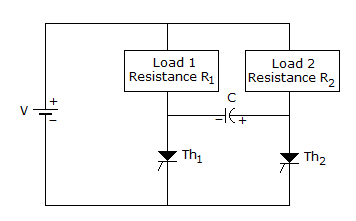# Electronics and Communication Engineering - Power Electronics

### Exercise :: Power Electronics - Section 5

36.

In a single phase full wave ac regulator using two thyristors only, the gate circuits of the two thyristors

 A. must be isolated B. may or may not be isolated C. should be preferably, isolated D. should not be isolated

Explanation:

No answer description available for this question. Let us discuss.

37.

The circuit in figure is forA. resonant commutation B. line commutation C. auxiliary commutation D. complementary commutation

Explanation:

No answer description available for this question. Let us discuss.

38.

A fully controlled bridge converter is feeding an RLE load. For some circuit conditions firing angle is 47°. If connections of battery are reversed, the firing angle is likely to be

 A. 47° B. less than 47° C. more than 47° D. either more or less than 47°

Explanation:

No answer description available for this question. Let us discuss.

39.

In a single phase half wave converter feeding resistive load and full wave converter (M - 2 connection) feeding a resistive load the circuit conditions are similar. The firing angle is a. Then the waveshapes of voltages across thyristors

 A. are similar B. are not similar C. may be similar or dissimilar D. are similar only for some values of a

Explanation:

No answer description available for this question. Let us discuss.

40.

In a 3 phase full converter feeding a highly inductive load, the average load current is 150 A. The peak current through thyristor is

 A. 150 A B. 75 A C. 50 A D. 300 A